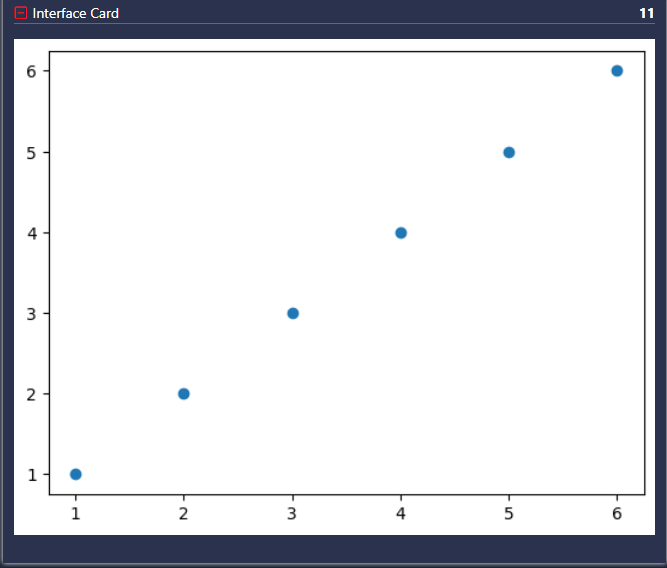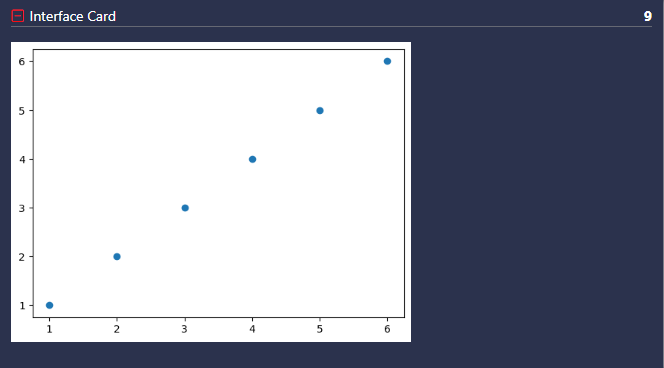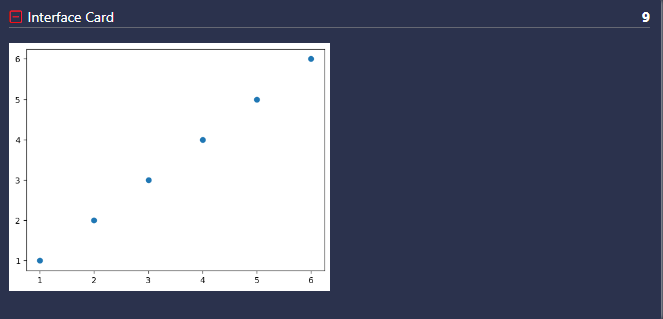# Overview

The Graph Output `@output graph`is used to output a matplotlib figure to an output card.# Usage

``````fig = plt.figure()
x = np.array([1,2,3,4,5,6])
y = np.array([1,2,3,4,5,6])
plt.scatter(x, y)
# @output graph data=fig
``````

# Named Arguments

Named Argument

Possible Values

Purpose

size

Custom Pixel Size (eg. "50x100, 50%x*,500x*")

Specifies the percentage width and the height of the output card that it occupies

# Examples

### Displaying Variable A Graph Output

``````fig = plt.figure()
x = np.array([1,2,3,4,5,6])
y = np.array([1,2,3,4,5,6])
plt.scatter(x, y)
# @output graph data=fig
``````### Displaying Variable As A Scatter Graph - Custom Pixel Size

``````fig = plt.figure()
x = np.array([1,2,3,4,5,6])
y = np.array([1,2,3,4,5,6])
plt.scatter(x, y)
# @output graph data=fig size=400x300
``````### Displaying Variable As A Scatter Graph - Custom Percentage Size

``````fig = plt.figure()
x = np.array([1,2,3,4,5,6])
y = np.array([1,2,3,4,5,6])
plt.scatter(x, y)
# @output graph data=fig size=50%x*
``````